# 从希卡文翻译谈谈 OCR 的简单实现## 希卡文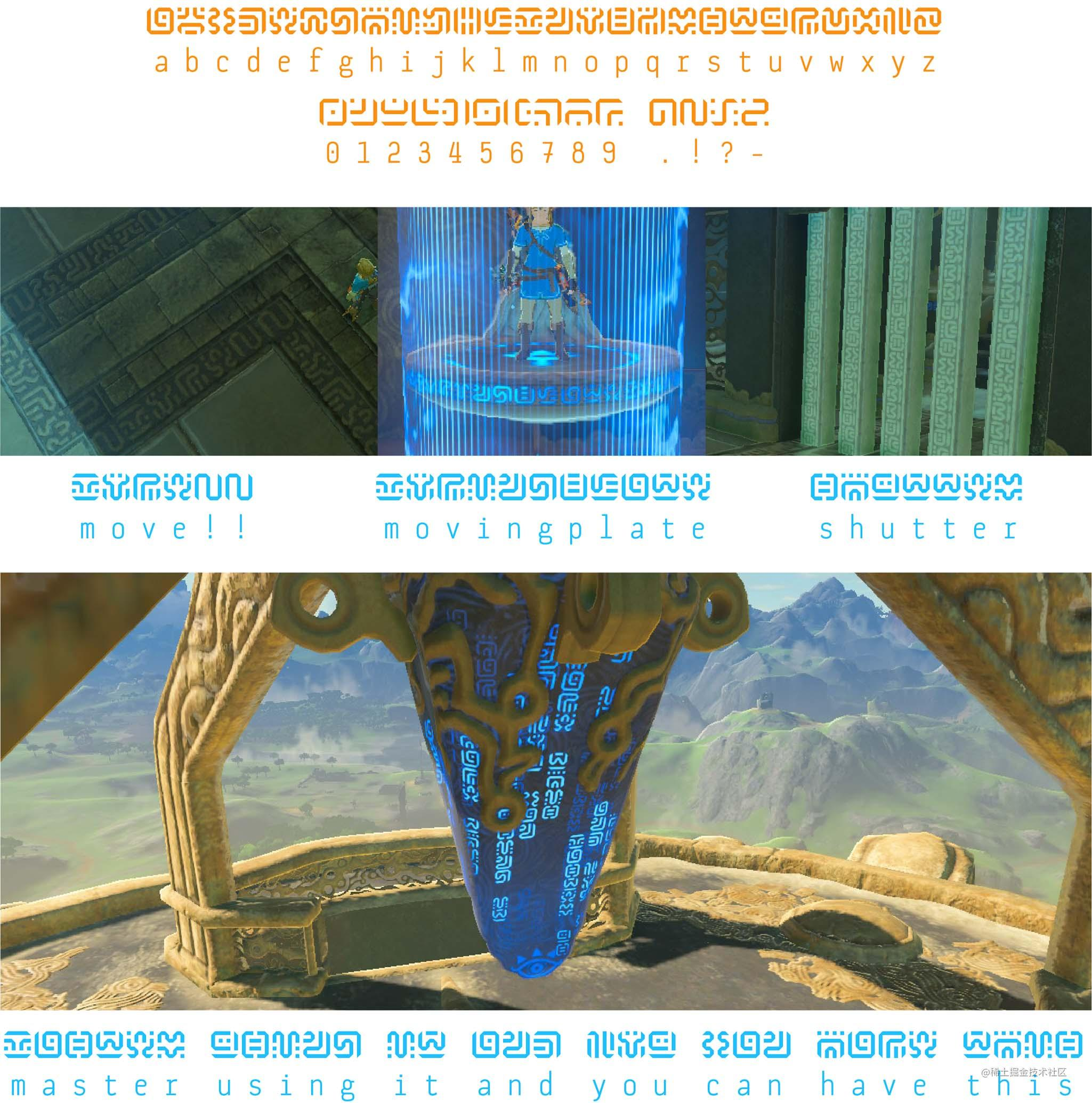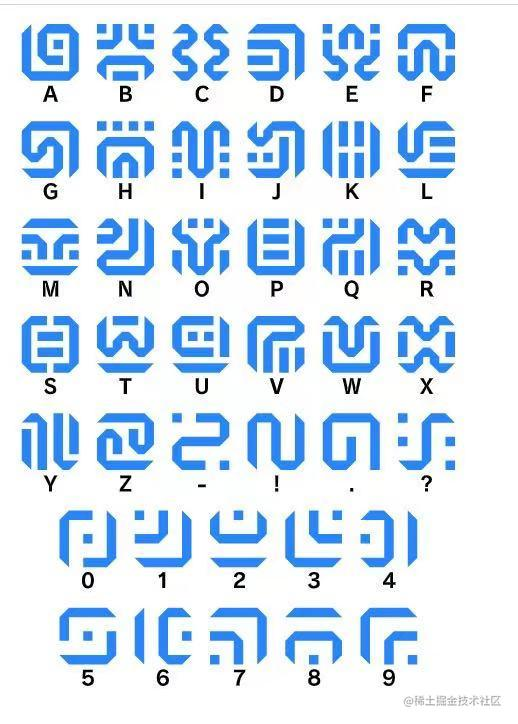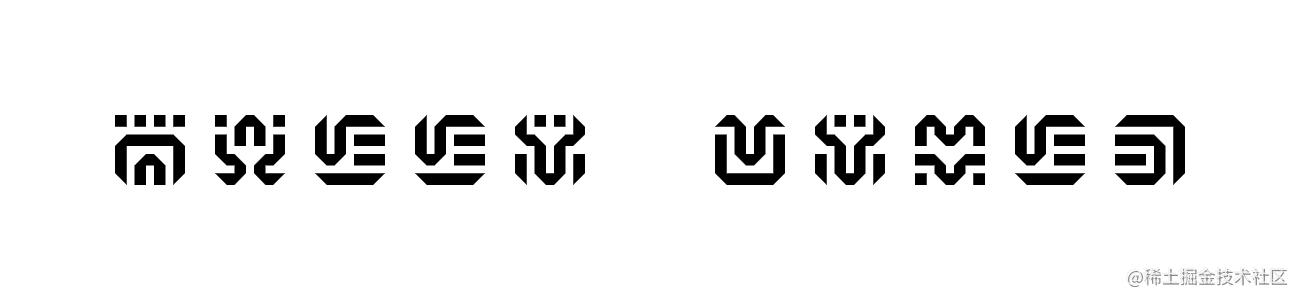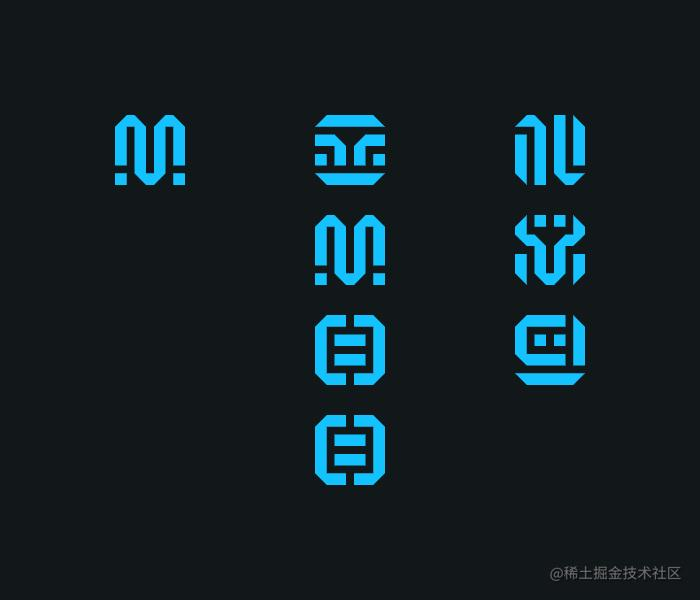## 图片二值化• 图片灰度处理
• 计算灰度图片的二值化阈值
• 图片二值化

### 图片灰度处理``````const canvasToGray = (canvas) => {
const ctx = canvas.getContext('2d');
const data = ctx.getImageData(0, 0, canvas.width, canvas.height);
const calculateGray = (r, g, b) => parseInt(r * 0.299 + g * 0.587 + b * 0.114);
const grayData = [];
for (let x = 0; x < data.width; x++) {
for (let y = 0; y < data.height; y++) {
const idx = (x + y * data.width) * 4;
const r = data.data[idx + 0];
const g = data.data[idx + 1];
const b = data.data[idx + 2];
const gray = calculateGray(r, g, b);
grayData.push(gray);
}
}
return grayData;
};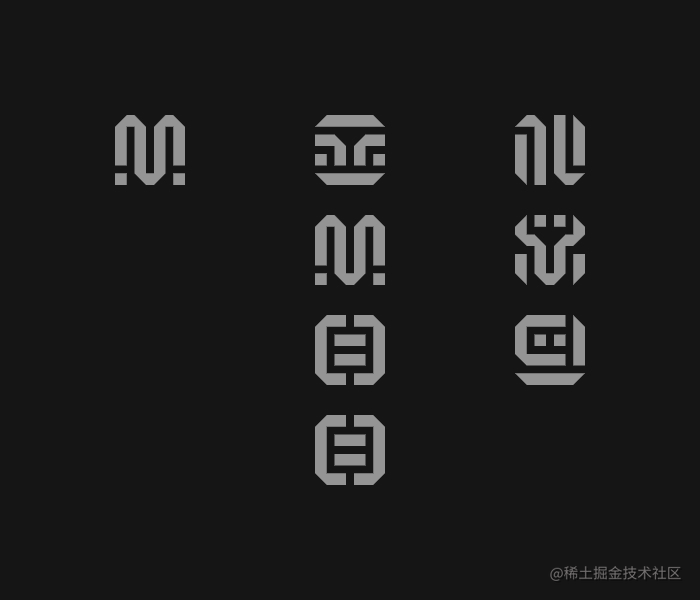### 二值化阈值

``````const average = (grayData) => {
let sum = 0;
for (let i = 0; i < grayData.length; i += 1) {
sum += data[i];
}
return sum / grayData.length;
};

``````const otsu = (grayData) => {
let ptr = 0;
// 记录0-256每个灰度值的数量，初始值为 0
let histData = Array(256).fill(0);
let total = grayData.length;

while (ptr < total) {
let h = grayData[ptr++];
histData[h]++;
}
// 总数(灰度值x数量)
let sum = 0;
for (let i = 0; i < 256; i++) {
sum += i * histData[i];
}
// 背景（小于阈值）的数量
let wB = 0;
// 前景（大于阈值）的数量
let wF = 0;
// 背景图像（灰度x数量）总和
let sumB = 0;
// 存储最大类间方差值
let varMax = 0;
// 阈值
let threshold = 0;

for (let t = 0; t < 256; t++) {
// 背景（小于阈值）的数量累加
wB += histData[t];
if (wB === 0) continue;
// 前景（大于阈值）的数量累加
wF = total - wB;
if (wF === 0) break;
// 背景（灰度x数量）累加
sumB += t * histData[t];

// 背景（小于阈值）的平均灰度
let mB = sumB / wB;
// 前景（大于阈值）的平均灰度
let mF = (sum - sumB) / wF;
// 类间方差
let varBetween = wB * wF * (mB - mF) ** 2;

if (varBetween > varMax) {
varMax = varBetween;
threshold = t;
}
}

return threshold;
};

### 图片二值化

``````const binaryzationOutput = (originCanvas, threshold) => {
const ctx = originCanvas.getContext('2d');
const imageData = ctx.getImageData(0, 0, originCanvas.width, originCanvas.height);
const { width, height, data } = imageData;
// 第一像素的值即为背景色值
const head = (data + data + data) / 3 | 0;
// 如果背景颜色大于阈值，则背景与文字的颜色的值则需要调换
const color = head > threshold
? { foreground: 0, background: 255 }
: { foreground: 255, background: 0 };
for (let x = 0; x < width; x++) {
for (let y = 0; y < height; y++) {
const idx = (x + y * width) * 4;
const avg = (data[idx] + data[idx + 1] + data[idx + 2]) / 3 | 0;
const v = avg > threshold ? color.foreground : color.background;
data[idx] = v;
data[idx + 1] = v;
data[idx + 2] = v;
data[idx + 3] = 255;
}
}
ctx.putImageData(imageData, 0, 0);
return originCanvas.toDataURL();
}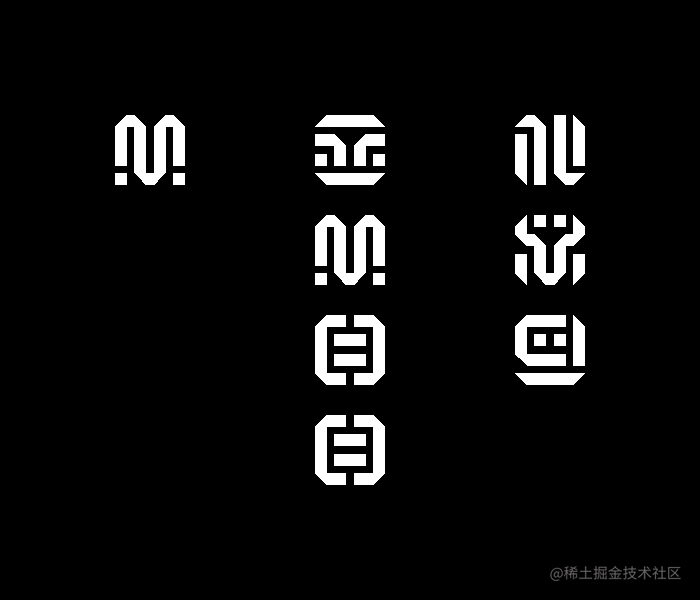## 文字切割

• 从上到下扫描图片每一行像素值，切割出文字所在的行
• 从左到右扫描文字行每一列像素值，切割出单个文字

### 切割行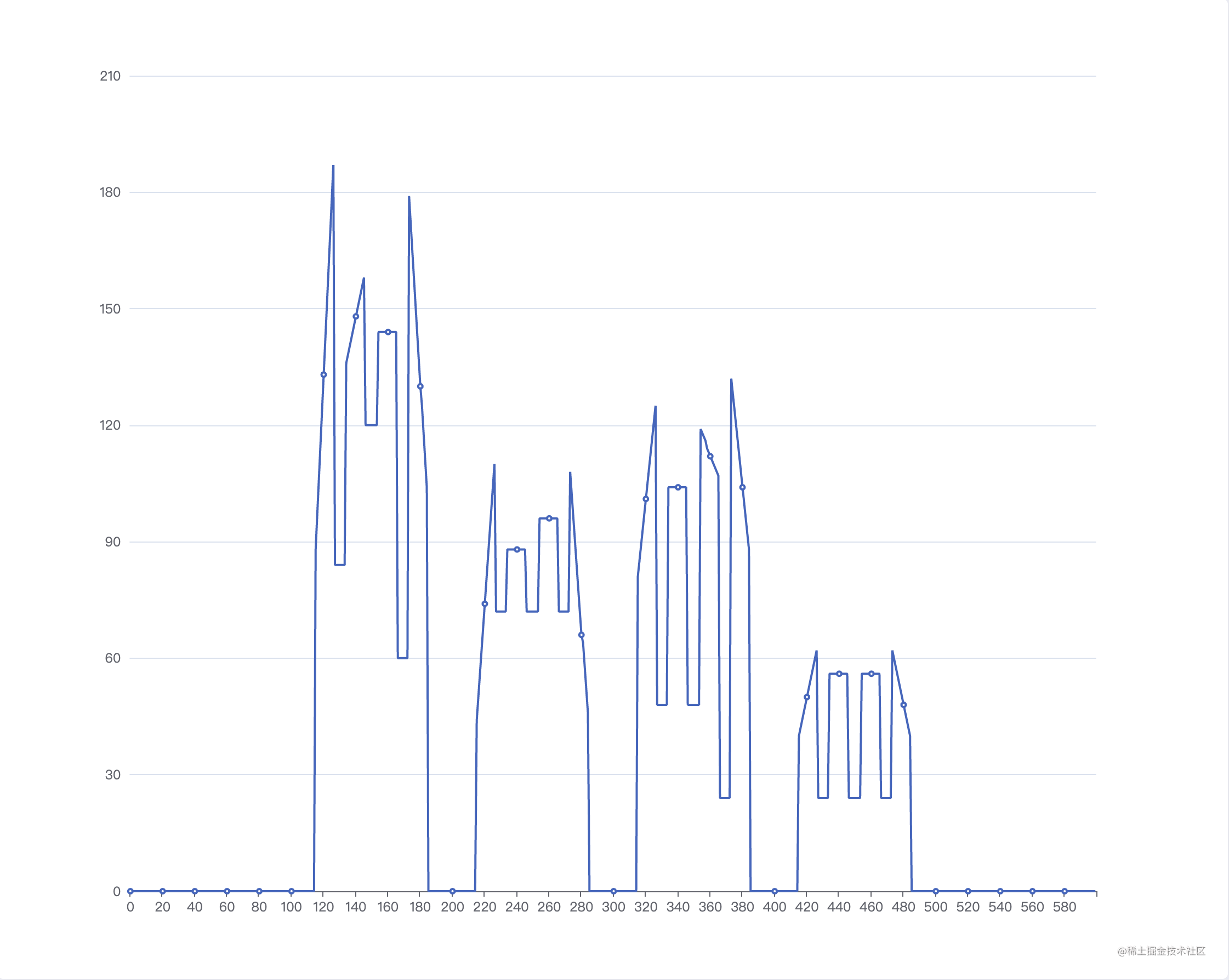### 切割文字（切割列）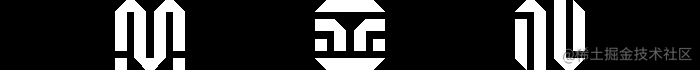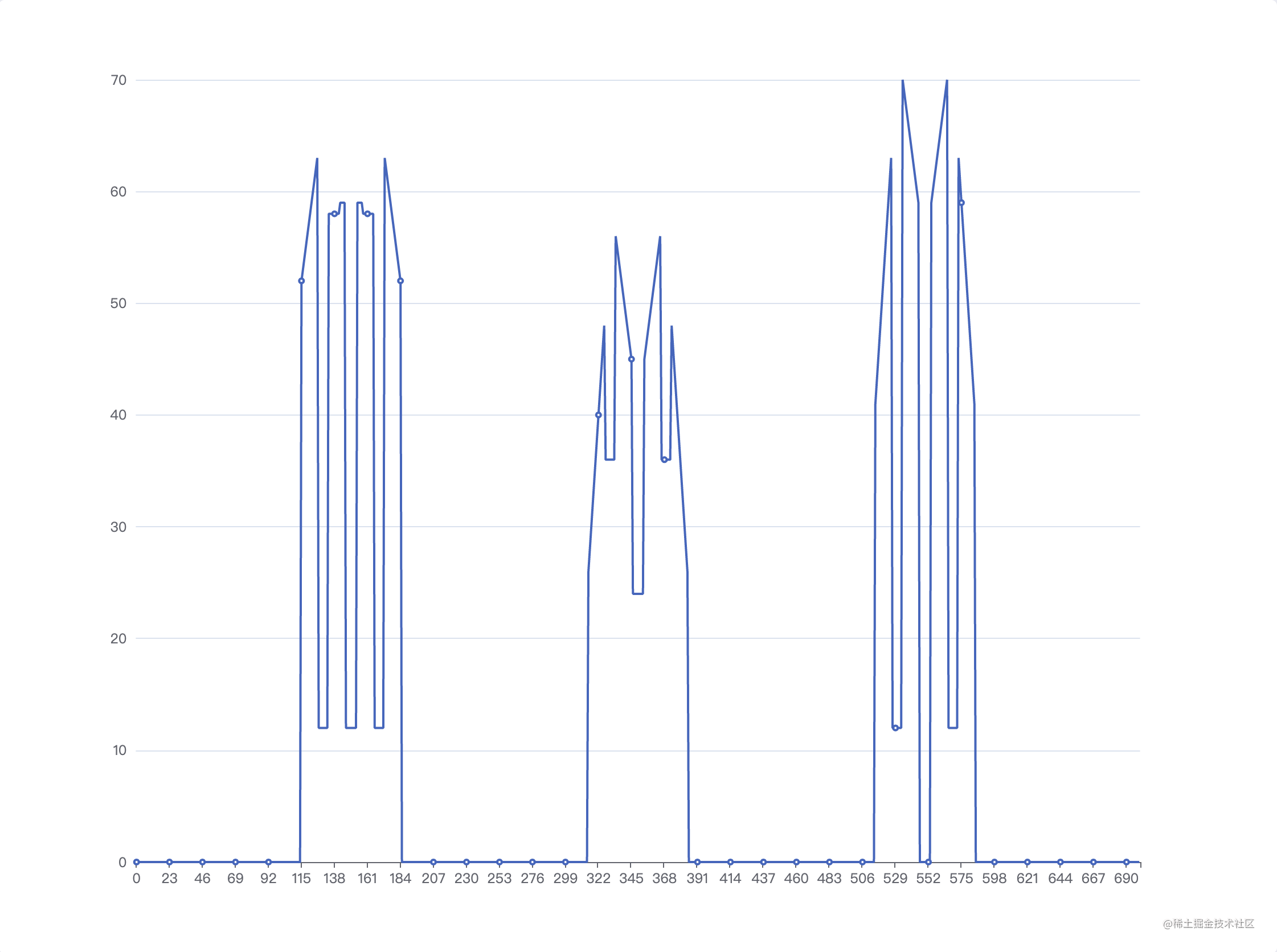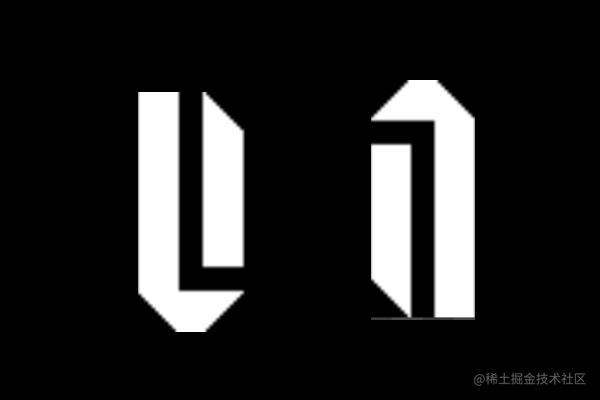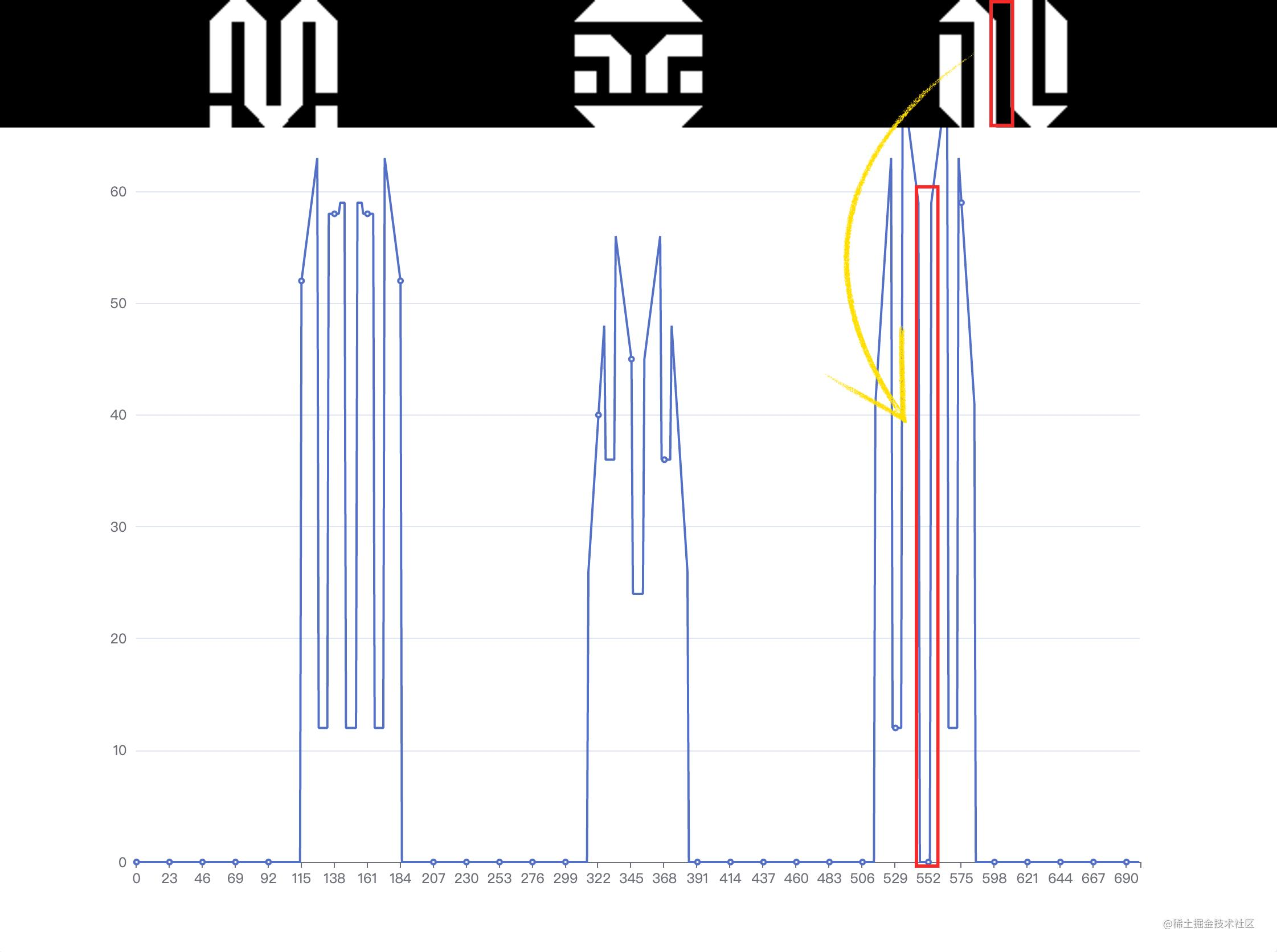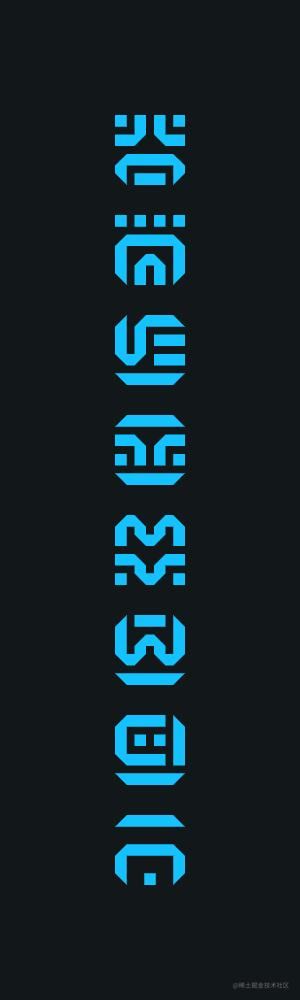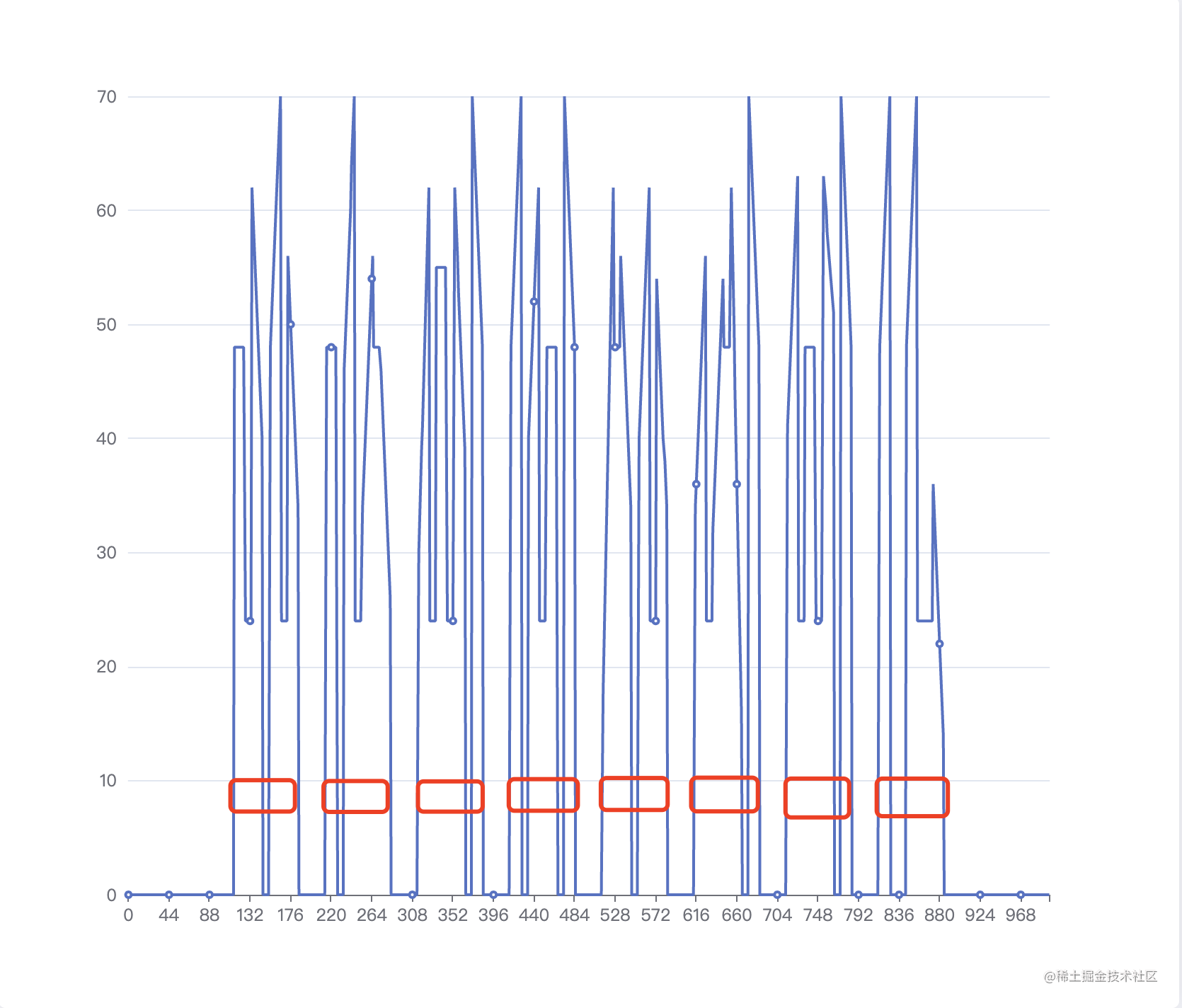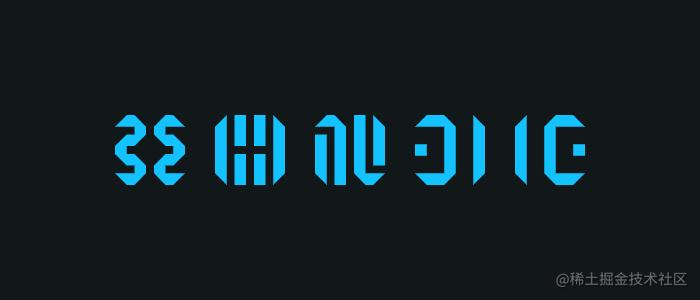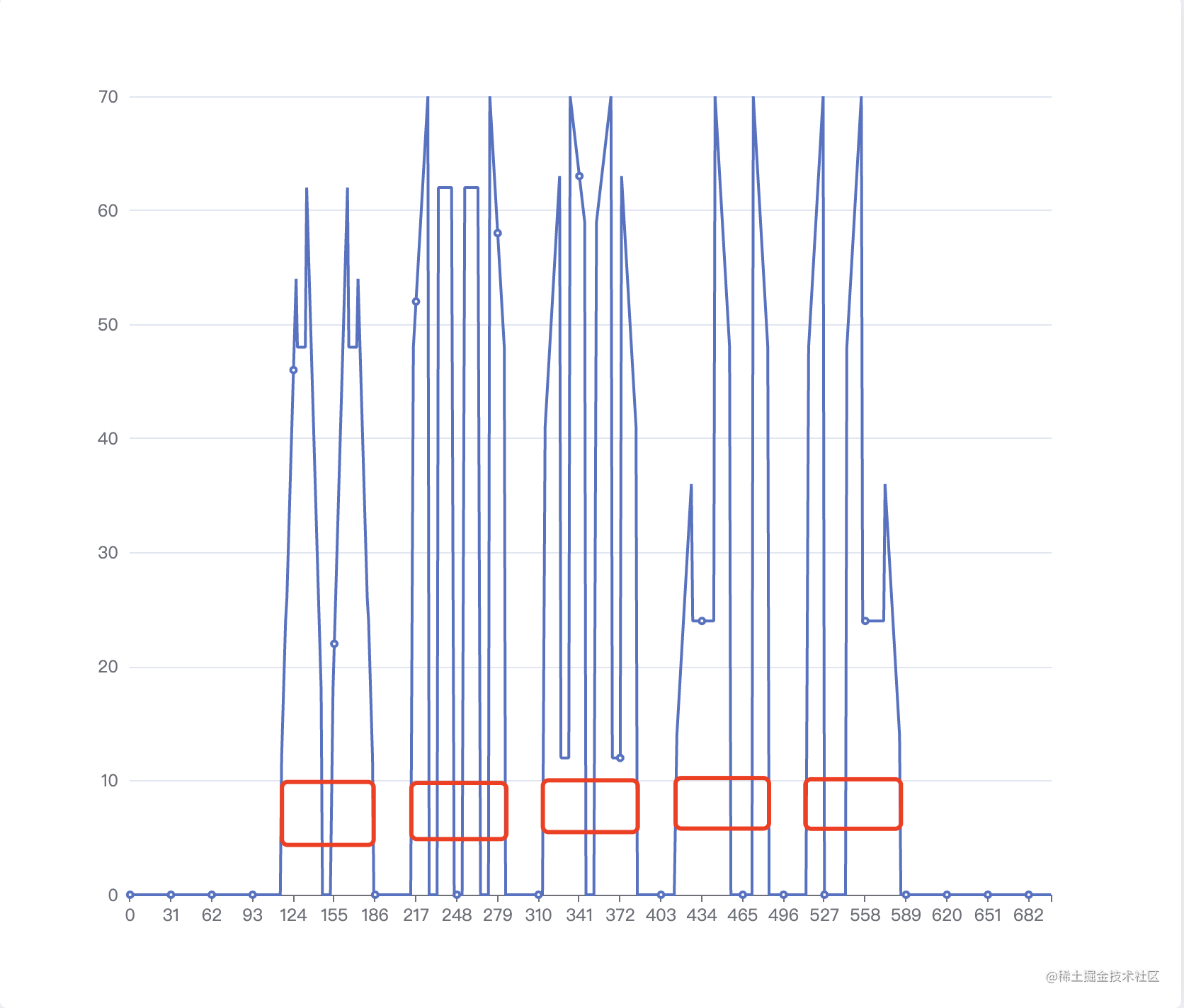``````// 横纵向扫描
function countPixel(imageData, isRow = false) {
const { width, height, data } = imageData;
const offsets = [0, 1, 2];
// 背景色
const head = offsets.map((i) => data[i]);
const pixel = [];
if (isRow) {
// 从上至下，横向扫描
for (let i = 0; i < height; i++) {
let count = 0;
for (let j = 0; j < width; j++) {
const index = (i * width + j) * 4;
const isEqual = offsets.every(
(offset) => head[offset] === data[index + offset]
);
count += isEqual ? 0 : 1;
}
pixel.push(count);
}
} else {
// 从左到右，纵向扫描
for (let i = 0; i < width; i++) {
let count = 0;
for (let j = 0; j < height; j++) {
const index = (j * width + i) * 4;
const isEqual = offsets.every(
(offset) => head[offset] === data[index + offset]
);
count += isEqual ? 0 : 1;
}
pixel.push(count);
}
}
return pixel;
}

// 拆分文字与背景区间
function countRanges(counts) {
const groups = [];
let foreground = 0;
let background = 0;
counts.forEach((count) => {
if (count) {
foreground += 1;
if (background) {
groups.push({ background: true, value: background });
background = 0;
}
} else {
background += 1;
if (foreground) {
groups.push({ foreground: true, value: foreground });
foreground = 0;
}
}
});
if (foreground) {
groups.push({ foreground: true, value: foreground });
}
if (background) {
groups.push({ background: true, value: background });
}
return groups;
}

// 获取文字内容的最大区间
function getMaxRange(data) {
return data.reduce((max, it) => {
if (it.foreground) {
return Math.max(max, it.value);
}
return max;
}, 0);
}

``````const imageData = {};
// 逐行扫描
const rowsRanges = countRanges(countPixel(imageData, true));
// 逐列扫描
const colsRanges = countRanges(countPixel(imageData, false));

// 计算横纵像素分布得出字体内容的大小（字体正方形区域）
const fontRange = Math.max(
getMaxRange(rowsRanges),
getMaxRange(colsRanges)
);

``````// 合并结构分离的文字区间
function mergeRanges(data, size) {
const merge = [];
// chunks 用来保存小于标准文字大小区域
let chunks = [];
data.forEach((item) => {
if (chunks.length) {
chunks.push(item);
const value = chunks.reduce((sum, chunk) => sum + chunk.value, 0);
// 当前换成的区域大小大于或接近标准文字大小则合并成一块
if (value >= size || Math.pow(value - size, 2) < 4) {
merge.push({
foreground: true,
value,
});
chunks = [];
}
return;
}
// 区域内容小于标准文字大小是推入 chunks
if (item.foreground && item.value < size) {
chunks = [item];
return;
}
merge.push(item);
});
return merge;
}

``````[
{
"background": true,
"value": 115
},
{
"foreground": true,
"value": 70
},
{
"background": true,
"value": 30
},
{
"foreground": true,
"value": 70
},
{
"background": true,
"value": 30
},
{
"foreground": true,
"value": 70
},
{
"background": true,
"value": 30
},
{
"foreground": true,
"value": 70
},
{
"background": true,
"value": 115
}
]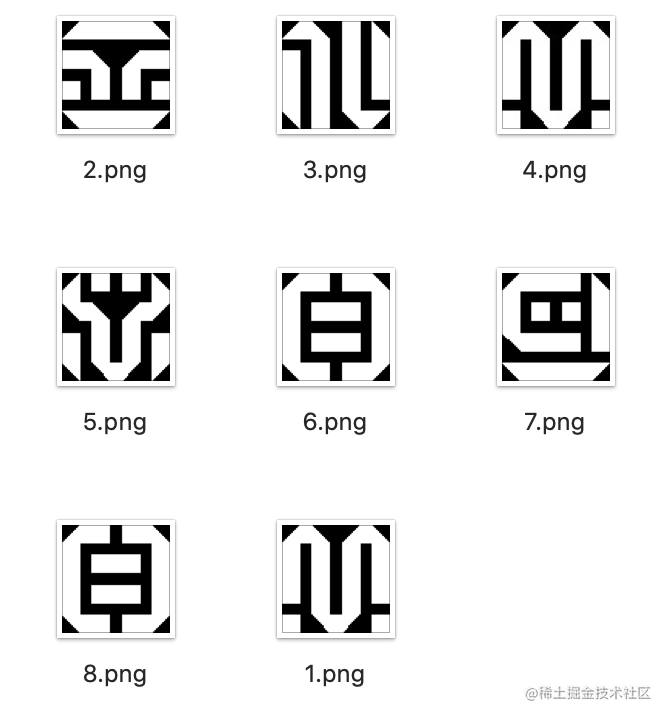## 相似图片检测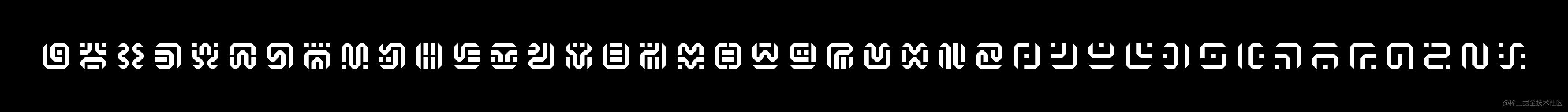``````const binaryzationHash = (originCanvas, threshold) => {
const ctx = originCanvas.getContext('2d');
const imageData = ctx.getImageData(0, 0, originCanvas.width, originCanvas.height);
const { width, height, data } = imageData;
// 第一像素的值即为背景色值
const head = (data + data + data) / 3 | 0;
// 如果背景颜色大于阈值，则背景与文字的颜色的值则需要调换
const color = head > threshold
? { foreground: 0, background: 255 }
: { foreground: 255, background: 0 };
const hash = [];
for (let x = 0; x < width; x++) {
for (let y = 0; y < height; y++) {
const idx = (x + y * width) * 4;
const avg = (data[idx] + data[idx + 1] + data[idx + 2]) / 3 | 0;
const v = avg > threshold ? color.foreground : color.background;
hash.push(v ? 1 : 0);
}
}
return hash;
}

``````const hammingDistance = (hash1, hash2) => {
let count = 0;
hash1.forEach((it, index) => {
count += it ^ hash2[index];
});
return count;
};

• 将比较的图片都缩小成 8 x 8
• 图片灰度化处理
• 计算二值化阈值
• 图片二值化计算图片哈希
• 比较两张图片哈希的汉明距离

1. 40 个标准图片统一缩小成 8 x 8 并生成对应的图片哈希
2. 切割出的文字图片统一缩小成 8 x 8 并生成对应的图片哈希
3. 切割出文字哈希逐个与 40 个标准图片哈希比较，挑选出差异（相似度最高的）最小就是目标字母

``````async function createImageFingerprints(image) {
const contents = splitImage(image);
return contents.map(({ canvas, ...args }) => {
// 统一缩小到 8 像素
const imageData = resizeCanvas(canvas, 8);
const hash = binaryzationOutput(imageData);
return {
...args,
hash,
};
});
}

// 生成标准字符指纹
function createSymbols(fingerprints) {
const WORDS = 'abcdefghijklmnopqrstuvwxyz0123456789.-!?';
return fingerprints.map((it, index) => {
return {
name: WORDS[index],
value: it.hash,
};
});
}

// 匹配出最相似的字符
function mapSymbols(fingerprints, symbols) {
return fingerprints.map(({ hash, ...position }) => {
const isEmpty = hash.every((v:) => v === hash);
if (isEmpty) {
return ' ';
}
let diff = Number.MAX_SAFE_INTEGER;
let word = '*';
symbols.forEach((symbol) => {
const distance = hammingDistance(hash, symbol.value);
// 汉明距离大于标识相似度偏差较大排除
if (distance < diff) {
diff = distance;
word = symbol.name;
}
});
return {
...position,
word,
diff,
};
});
}

``````/**
* @param imageUrl 解析的图片
* @param mapUrl 标准字符图片
*/
export async function readMetaInfo(imageUrl, mapUrl) {
const mapImage = await loadImage(mapUrl);
const mapImageFingerprints = await createImageFingerprints(mapImage, false);
const symbols = createSymbols(mapImageFingerprints);
const readImageFingerprints = await createImageFingerprints(
true
);
const results = mapSymbols(readImageFingerprints, symbols);
console.log(results);
}

## 其他